• 两个图片提取的两个特征向量，二维结构，结构如下： array([[ 2.62957041e+00, 7.46614219e-05, 2.37797423e-05, ..., -4.94050192e-04, 2.06032040e-03, 4.94050192e-04], [-1.05751487e+00, 0.00000000e+00...机器学习
• ## Python计算图片之间的相似度

万次阅读 多人点赞 2018-11-17 16:39:05
把图片表示成一个向量，通过计算向量之间的余弦距离来表征张图片的相似度 # -*- coding: utf-8 -*- # !/usr/bin/env python # @Time : 2018/11/17 14:52 # @Author : xhh # @Desc : 余弦相似度计算 # @File ...
1.cosin相似度（余弦相似度）
把图片表示成一个向量，通过计算向量之间的余弦距离来表征两张图片的相似度

# -*- coding: utf-8 -*-
# !/usr/bin/env python
# @Time    : 2018/11/17 14:52
# @Author  : xhh
# @Desc    : 余弦相似度计算
# @File    : difference_image_consin.py
# @Software: PyCharm
from PIL import Image
from numpy import average, dot, linalg

# 对图片进行统一化处理
def get_thum(image, size=(64,64), greyscale=False):
# 利用image对图像大小重新设置, Image.ANTIALIAS为高质量的
image = image.resize(size, Image.ANTIALIAS)
if greyscale:
# 将图片转换为L模式，其为灰度图，其每个像素用8个bit表示
image = image.convert('L')
return image

# 计算图片的余弦距离
def image_similarity_vectors_via_numpy(image1, image2):
image1 = get_thum(image1)
image2 = get_thum(image2)
images = [image1, image2]
vectors = []
norms = []
for image in images:
vector = []
for pixel_tuple in image.getdata():
vector.append(average(pixel_tuple))
vectors.append(vector)
# linalg=linear（线性）+algebra（代数），norm则表示范数
# 求图片的范数？？
norms.append(linalg.norm(vector, 2))
a, b = vectors
a_norm, b_norm = norms
# dot返回的是点积，对二维数组（矩阵）进行计算
res = dot(a / a_norm, b / b_norm)
return res

image1 = Image.open('../dataset/100002.png')
image2 = Image.open('../dataset/100001.png')
cosin = image_similarity_vectors_via_numpy(image1, image2)
print('图片余弦相似度',cosin)
运行结果：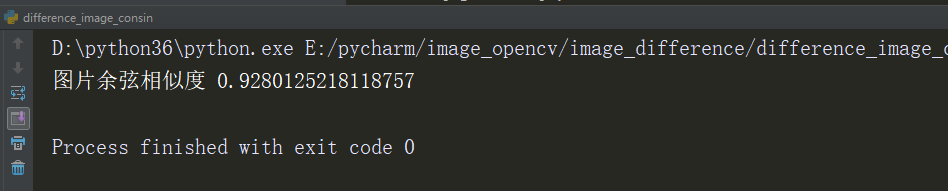该方法运算量较大，速度明显比ssim方法慢，但如果不计较快慢的话感受结果比ssim靠谱

2.利用SSIM（结构相似度度量）计算图片的相似度
是一种全参考的图像质量评价指标，分别从亮度、对比度、结构三个方面度量图像相似性。
SSIM取值范围[0, 1]，值越大，表示图像失真越小。
在实际应用中，可以利用滑动窗将图像分块，令分块总数为N，考虑到窗口形状对分块的影响，采用高斯加权计算每一窗口的均值、方差以及协方差，然后计算对应块的结构相似度SSIM，最后将平均值作为两图像的结构相似性度量，即平均结构相似性SSIM
# -*- coding: utf-8 -*-
# !/usr/bin/env python
# @Time    : 2018/11/17 14:26
# @Author  : xhh
# @Desc    : 结构相似度量，计算图片之间的相似度
# @File    : difference_image_ssim.py
# @Software: PyCharm
from skimage.measure import compare_ssim
import numpy as np

# 读取图片
img2 = np.resize(img2, (img1.shape, img1.shape, img1.shape))
print(img1.shape)
print(img2.shape)
ssim =  compare_ssim(img1, img2, multichannel = True)
print(ssim)
scipy.misc import imread成功了，最后又改成了opencv又好了，有点懵逼了

结果：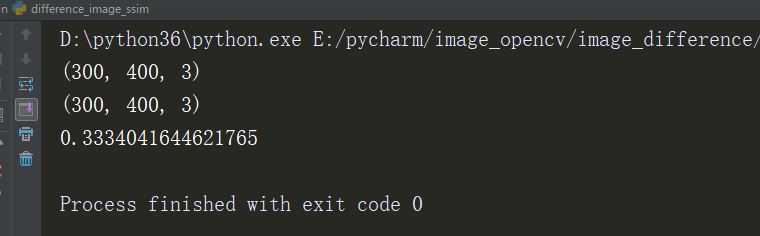该方法通常用来衡量一张图片压缩后的失真度，很少用来计算两图的相似度。
3.通过直方图计算
# -*- coding: utf-8 -*-
# !/usr/bin/env python
# @Time    : 2018/11/17 16:04
# @Author  : xhh
# @Desc    : 通过直方图计算图片的相似度
# @File    : difference_image_hist.py
# @Software: PyCharm
from PIL import Image

# 将图片转化为RGB
def make_regalur_image(img, size=(64, 64)):
gray_image = img.resize(size).convert('RGB')
return gray_image

# 计算直方图
def hist_similar(lh, rh):
assert len(lh) == len(rh)
hist = sum(1 - (0 if l == r else float(abs(l-r))/max(l,r))for l, r in zip(lh, rh))/len(lh)
return hist

# 计算相似度
def calc_similar(li, ri):
calc_sim = hist_similar(li.histogram(), ri.histogram())
return calc_sim

if __name__ == '__main__':
image1 = Image.open('../dataset/100002.png')
image1 = make_regalur_image(image1)
image2 = Image.open('../dataset/100001.png')
image2 = make_regalur_image(image2)
print("图片间的相似度为",calc_similar(image1, image2))


运行结果：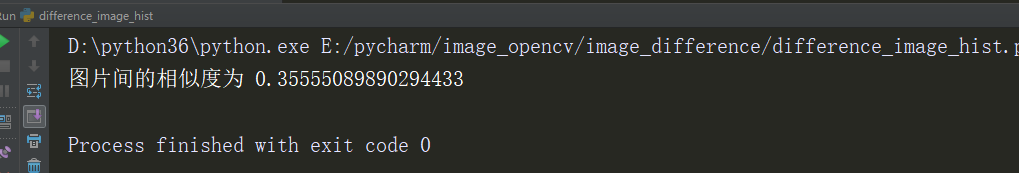直方图过于简单，只能捕捉颜色信息的相似性，捕捉不到更多的信息。只要颜色分布相似，就会判定二者相似度较高。
所以本菜鸟利用之前做验证码时的用灰度图和彩色图进行了对比：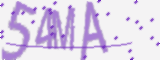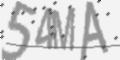运行的结果为：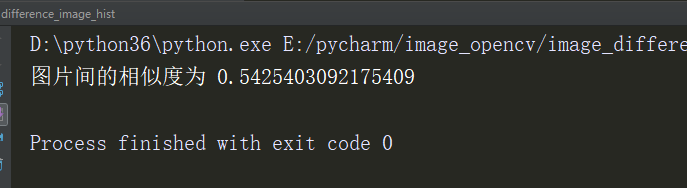结果为0.54，有点差强人意了。。
4.还有通过感知哈希算法（或者也称为图片指纹的形式的，嗯，我是这样子理解的）
具体的代码看之前的文章：https://blog.csdn.net/weixin_39121325/article/details/84174876
。。。。占时就这些了，还有的遇到了在更新吧
大家可以关注我和我小伙伴的公众号~~~这里有我和我的小伙伴不定时的更新一些python技术资料哦！！大家也可以留言，讨论一下技术问题，希望大家多多支持，关注一下啦，谢谢大家啦~~展开全文• def learn_cosine_similarity(): a = [[1, 3, 2], [2, 2, 1]] from sklearn.metrics.pairwise import cosine_similarity from sklearn.metrics.pairwise import pairwise_distances print('...python
• 计算两个句子向量 2、np.linalg.norm求 向量模长(一范二范) 3、python列表转换为矩阵 1、Python求余弦相似度 在非直角三角形中,余弦定理的公式是: 在向量表示的三角形中，假设a向量是（x1, y1），b向量是(x2,...
目录
1、Python求余弦相似度
计算两个句子向量
2、np.linalg.norm 求 向量模长(一范二范)
3、python列表转换为矩阵

1、Python求余弦相似度
在非直角三角形中,余弦定理的公式是: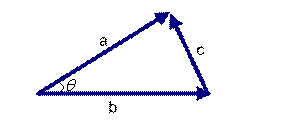在向量表示的三角形中，假设a向量是（x1, y1），b向量是(x2, y2)，那么可以将余弦定理改写成下面的形式：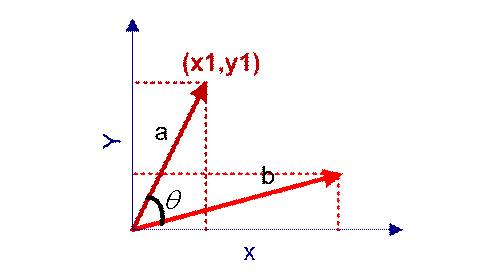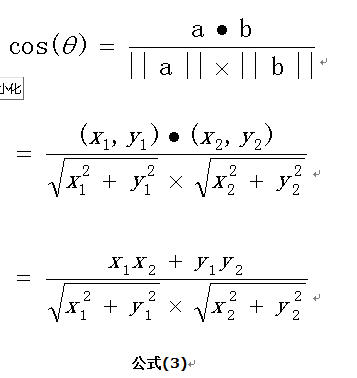扩展，如果向量a和b不是二维而是n维，上述余弦的计算法仍然正确。假定a和b是两个n维向量，则a与b的夹角 的余弦等于：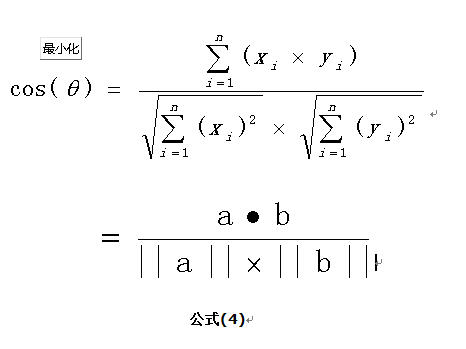计算两个句子向量
句子A：(1，1，2，1，1，1，0，0，0)
和句子B：(1，1，1，0，1，1，1，1，1)的向量余弦值来确定两个句子的相似度。
根据：计算过程及（通用）代码如下：1、调用sklearn
from sklearn.metrics.pairwise import cosine_similarity

a = [[1, 3, 2], [2, 2, 1]]
print(cosine_similarity(a))
2、方法1，常规算法，计算较慢
def cos_sim(s1_cut_code, s2_cut_code):
# 计算余弦相似度
sum = 0
sq1 = 0
sq2 = 0
for i in range(len(s1_cut_code)):
sum += s1_cut_code[i] * s2_cut_code[i]
sq1 += pow(s1_cut_code[i], 2)
sq2 += pow(s2_cut_code[i], 2)

try:
result = round(float(sum) / (math.sqrt(sq1) * math.sqrt(sq2)), 3)
except ZeroDivisionError:
result = 0.0
#     print("余弦相似度为：%f"%result)
return result
方法2 计算较快
def norm(vector):
return math.sqrt(sum(x * x for x in vector))

def cosine_similarity(vec_a, vec_b):
norm_a = norm(vec_a)
norm_b = norm(vec_b)
dot = sum(a * b for a, b in zip(vec_a, vec_b))
return dot / (norm_a * norm_b)
3、稀疏矩阵
def computer_simMatrix(c):
"""
稀疏矩阵求相似度
"""
return c.dot(c.T)/sparse.linalg.norm(c,axis=1).reshape((-1,1)).dot(sparse.linalg.norm(c,axis=1).reshape((1,-1)))

# 返回一个矩阵，上三角和下三角是对称的，维度=len(c)*len(c)

2、np.linalg.norm 求 向量模长(一范二范)
x_norm=np.linalg.norm(x, ord=None, axis=None, keepdims=False)
①x: 表示矩阵（也可以是一维）
②ord：范数类型
向量的范数：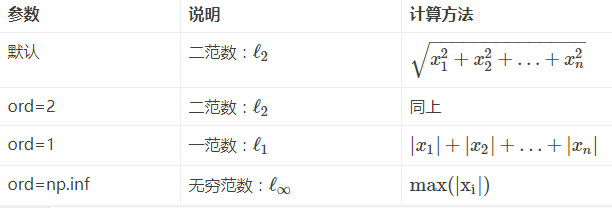矩阵的范数：
ord=1：列和的最大值
ord=2：|λE-ATA|=0，求特征值，然后求最大特征值得算术平方根
ord=∞：行和的最大值
③axis：处理类型
axis=1表示按行向量处理，求多个行向量的范数
axis=0表示按列向量处理，求多个列向量的范数
axis=None表示矩阵范数。
④keepding：是否保持矩阵的二维特性
True表示保持矩阵的二维特性，False相反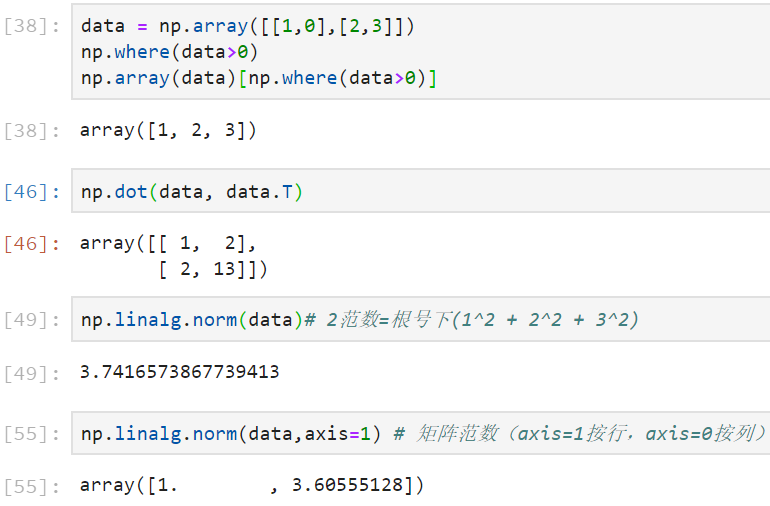# 求向量的三种范式，代码如下：
A = np.array([[3,4],[-6,5]])

ret = np.linalg.norm(A,ord=None) # 对象是矩阵，求矩阵元素平方和开放
print(ret)

ret = np.linalg.norm(A,ord=None,axis=1) # 对象是逐行，求每行元素平方和开放
print(ret)

ret = np.linalg.norm(A,ord=None,axis=0)  # 对象是逐列，求每列元素平方和开放
print(ret)
# 对象是矩阵，求矩阵中逐行元素绝对值和的最大值
ret = np.linalg.norm(A,ord=np.Inf)
print(ret)

ret = np.linalg.norm(A,ord=np.Inf,axis=1) # 对象是逐行，求每行元素绝对值和的最大值
print(ret)

ret = np.linalg.norm(A,ord=np.Inf,axis=0) # 对象是逐列，求每列元素绝对值的最大值
print(ret)

# 对象是矩阵，求矩阵中逐行元素绝对值和的最小值
ret = np.linalg.norm(A,ord=-np.Inf)
print(ret)

ret = np.linalg.norm(A,ord=-np.Inf,axis=1) # 对象是逐行，求行的元素绝对值的最小值
print(ret)

ret = np.linalg.norm(A,ord=-np.Inf,axis=0) # 对象是逐列，求列的元素绝对值的最小值
print(ret)
A = np.array([[3,-4,1],[-6,5,0]])

ret = np.linalg.norm(A,ord=0,axis=1) # 对象是逐行，求每行的非零元素个数
print(ret)

ret = np.linalg.norm(A,ord=0,axis=0) # 对象是逐列，求每列的非零元素个数
print(ret)
A = np.array([[100,4],[-6,5]])
ret = np.linalg.norm(A,ord=1)   # 对象是矩阵，求列的元素绝对值和的最大值
print(ret)

ret = np.linalg.norm(A,ord=1,axis=1)  # 对象是逐行，求行的元素绝对值和
print(ret)

ret = np.linalg.norm(A,ord=1,axis=0)    # 对象是逐列，求；列的元素绝对值和
print(ret)
A = np.array([[-4,3,1],[-6,5,-1]])
ret = np.linalg.norm(A,ord=-1)  # 对象是矩阵，求列的元素绝对值和最小值
print(ret)
ret = np.linalg.norm(A,ord=-1,axis=1) # 对象是逐行
print(ret)
ret = np.linalg.norm(A,ord=-1,axis=0)  # 对象是逐列
print(ret)
A = np.array([[-4,  3, 4],
[-6,  4, 3]])
#10.0015300174, 对象是矩阵，求所有元素平方和开方，所有元素平方和102，开方=10.0015300174
ret = np.linalg.norm(A, ord=2)
#[ 6.40312424  7.81024968]，对象是每行，求行的元素平方和开方
ret = np.linalg.norm(A, ord=2, axis=1)
#[ 7.21110255  5. 5.]，对象是每列，求列的元素平方和开方
ret = np.linalg.norm(A, ord=2, axis=0)

3、python列表转换为矩阵
#创建列表
>>> l=[] //然后用列表的insert函数将系数项插入到列表中去，最后将列表转换为矩阵
insert(“插入位置”，插入的内容对象）
>>> l.insert(0,[9,52,381])
>>> l
[[9, 52, 381]]
>>> l.insert(1,[52,381,3017])
>>> l
[[9, 52, 381], [52, 381, 3017]]
>>> l.insert(2,[381,3017,25317])
>>> l
[[9, 52, 381], [52, 381, 3017], [381, 3017, 25317]]
>>> a=np.mat(l)#这里将列表转换为矩阵
>>> a
matrix([[ 9, 52, 381],
[ 52, 381, 3017],
[ 381, 3017, 25317]])

>>> l=[]
>>> l.insert(0,)
>>> l.insert(1,)
>>> l.insert(2,)
>>> l
[, , ]
>>> b=np.mat(l)#将列表转换为矩阵
>>> b
matrix([[ 32],
[ 147],
])

scipy.sparse的一些整理 https://blog.csdn.net/qq_33466771/article/details/80304498
Scipy教程 - 线性代数库scipy.linalg http://blog.csdn.net/pipisorry/article/details/43277755
相似度算法之余弦相似度 https://blog.csdn.net/zz_dd_yy/article/details/51926305
python求向量的三种范式 https://blog.csdn.net/wangdong2017/article/details/81297654

展开全文• ## Python计算余弦相似度

千次阅读 多人点赞 2020-08-09 10:51:39
余弦相似度常用在文本分类、图片分类等应用中，来计算两个文本或两个图像之间的相似度。 本文主要介绍通过Python计算两个向量的余弦相似度
余弦相似度常用在文本分类、图片分类等应用中，来计算两个文本或两个图像之间的相似度。
如下图，向量

a

=

[

x

1

,

y

1

]

,

b

=

[

x

2

,

y

2

]

\boldsymbol a=[x_1,y_1],\boldsymbol b =[x_2,y_2]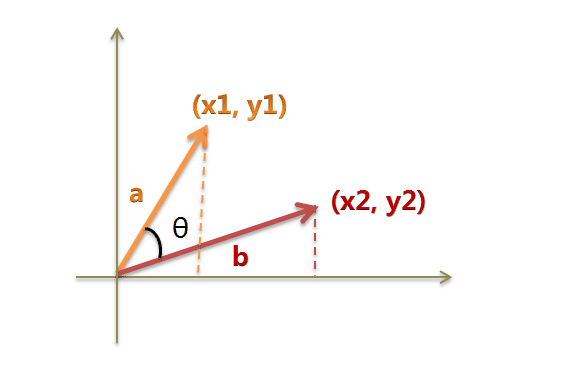s

i

m

(

a

,

b

)

=

c

o

s

θ

=

a

b

∣

a

∣

∣

b

∣

=

x

1

x

2

+

y

1

y

2

x

1

2

+

y

1

2

x

2

2

+

y

2

2

sim(a,b) = cos \theta = \frac {ab}{\mid a \mid \mid b \mid} = \frac {x_1x_2+y_1y_2}{\sqrt{x_1^2+y_1^2}\sqrt{x_2^2+y_2^2}}

对于

n

n

维向量

A

=

[

a

1

,

a

2

,

.

.

.

a

n

]

,

B

=

[

b

1

,

b

2

,

.

.

.

b

n

]

A=[a_1,a_2,...a_n],B=[b_1,b_2,...b_n]

，

s

i

m

(

A

,

B

)

=

A

B

∣

A

∣

∣

B

∣

=

∑

i

=

1

n

A

i

B

i

∑

i

=

1

n

A

i

2

∑

i

=

1

n

A

i

2

sim(A,B) = \frac {AB}{\mid A \mid \mid B \mid}= \frac {\sum_{i=1}^{n}{A_iB_i}}{\sqrt{\sum_{i=1}^{n}{A_i^2}}\sqrt{\sum_{i=1}^{n}{A_i^2}}}

余弦相似度的取值范围在-1到1之间。余弦值越接近1，也就是两个向量越相似，完全相同时数值为1；相反反向时为-1；正交或不相关是为0。
求余弦相似度需要用到np.linalg.norm 操作，来求向量的范式，默认是L2范式，等同于求向量的欧式距离。
import numpy as np
t1  = np.array([-0.4,0.8,0.5,-0.2,0.3])
t2  = np.array([-0.5,0.4,-0.2,0.7,-0.1])

def cos_sim(a, b):
a_norm = np.linalg.norm(a)
b_norm = np.linalg.norm(b)
cos = np.dot(a,b)/(a_norm * b_norm)
return cos
print(cos_sim(t1,t2))

输出：0.23612240736068565
展开全文• 余弦相似度，又称为余弦相似性，是通过计算两个向量的夹角余弦值来评估他们的相似度。余弦相似度将向量根据坐标值，绘制到向量空间中，如最常见的二维空间。 # -*- coding: utf-8 -*- import jieba import ...
余弦相似度，又称为余弦相似性，是通过计算两个向量的夹角余弦值来评估他们的相似度。余弦相似度将向量根据坐标值，绘制到向量空间中，如最常见的二维空间。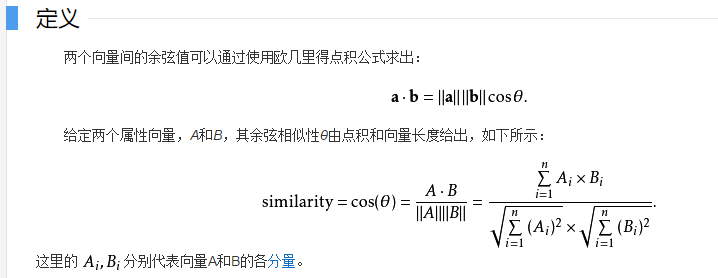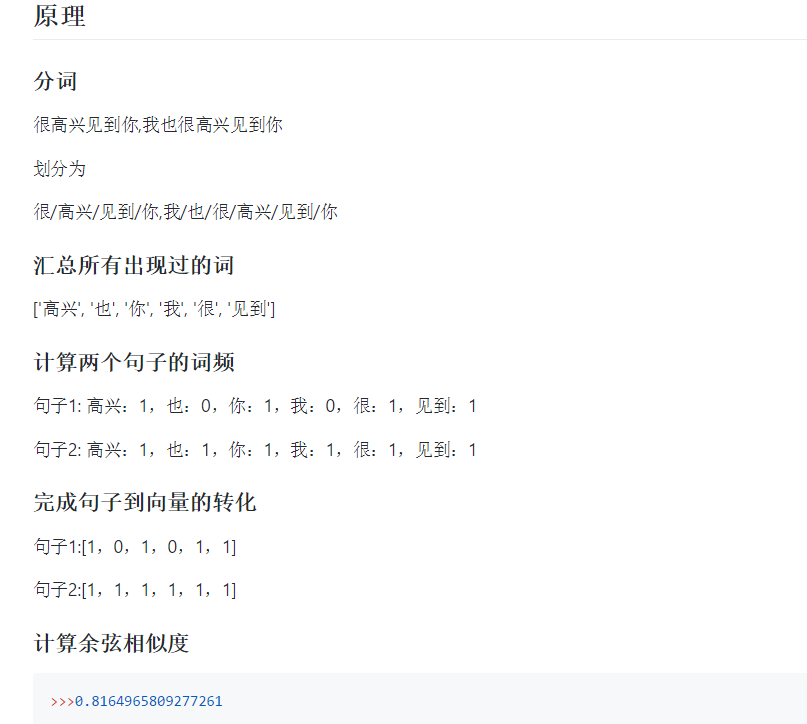github 参考链接：https://github.com/ZhanPwBibiBibi/CHlikelihood
# -*- coding: utf-8 -*-
import jieba
import numpy as np
import re

def get_word_vector(s1,s2):
"""
:param s1: 句子1
:param s2: 句子2
:return: 返回句子的余弦相似度
"""
# 分词
cut1 = jieba.cut(s1)
cut2 = jieba.cut(s2)
list_word1 = (','.join(cut1)).split(',')
list_word2 = (','.join(cut2)).split(',')

# 列出所有的词,取并集
key_word = list(set(list_word1 + list_word2))
# 给定形状和类型的用0填充的矩阵存储向量
word_vector1 = np.zeros(len(key_word))
word_vector2 = np.zeros(len(key_word))

# 计算词频
# 依次确定向量的每个位置的值
for i in range(len(key_word)):
# 遍历key_word中每个词在句子中的出现次数
for j in range(len(list_word1)):
if key_word[i] == list_word1[j]:
word_vector1[i] += 1
for k in range(len(list_word2)):
if key_word[i] == list_word2[k]:
word_vector2[i] += 1

# 输出向量
print(word_vector1)
print(word_vector2)
return word_vector1, word_vector2

def cos_dist(vec1,vec2):
"""
:param vec1: 向量1
:param vec2: 向量2
:return: 返回两个向量的余弦相似度
"""
dist1=float(np.dot(vec1,vec2)/(np.linalg.norm(vec1)*np.linalg.norm(vec2)))
return dist1

def filter_html(html):
"""
:param html: html
:return: 返回去掉html的纯净文本
"""
dr = re.compile(r'<[^>]+>',re.S)
dd = dr.sub('',html).strip()
return dd

if __name__ == '__main__':
s1="很高兴见到你"
s2="我也很高兴见到你"
vec1,vec2=get_word_vector(s1,s2)
dist1=cos_dist(vec1,vec2)
print(dist1)

运行结果：
Building prefix dict from the default dictionary ...
Prefix dict has been built succesfully.
[1. 1. 1. 1. 0. 0.]
[1. 1. 1. 1. 1. 1.]
0.8164965809277261

展开全文• 背景在计算相似度时，常常用到余弦夹角来判断相似度，Cosine（余弦相似度）取值范围[-1,1]，当两个向量的方向重合时夹角余弦取最大值1，当两个向量的方向完全相反夹角余弦取最小值-1，两个方向正交时夹角余弦取值为0...
• 1.余弦相似度可用来计算两个向量的相似程度 对于如何计算两个向量的相似程度问题，可以把这它们想象成空间中的两条线段，都是从原点（[0, 0, …]）出发，指向不同的方向。两条线段之间形成一个夹角，如果夹角为0度，...
• 可以把对文本内容的处理简化为向量空间中的向量运算，计算向量空间上的相似度，来表示文本语义上的相似度。 要使用word2vec工具就我所知有种方式，一种是从谷歌下载安装，需要使用linux环境；另一种是python中...文本处理 word2vec
• 计算相似度时，常常用到余弦夹角来判断相似度，Cosine（余弦相似度）取值范围[-1,1]，当两个向量的方向重合时夹角余弦取最大值1，当两个向量的方向完全相反夹角余弦取最小值-1，两个方向正交时夹角余弦取值为0。...线性代数
• 原标题：python文本相似度计算 ...两个向量计算相似度就很简单了，欧式距离、余弦相似度等等各种方法，只需要中学水平的数学知识。那么如何将文本表示成向量呢？词袋模型最简单的表示方法是词袋模型。把一篇文本想...
• 最近一直在研究NLP的文本相似度算法，本文将利用TF-IDF特征向量和Simhash指纹计算中文文本的相似度。 2、计算过程 准备测试数据 预处理读到的数据 加载数据到Map中 输入用户问题 利用TF特征向量和Simhash...tfidf
• 作者系浙江大学生物医学工程专业的硕士在读；专注于自然语言处理 iOS开发。 步骤 1、分词、去停用词 ...5、计算相似度 ...两篇中文文本，如何计算相似度？...两个向量计算相似度就很简单了，欧式距离、余弦相似度
• similarity 出来是对称矩阵，只需要取就ok了 print("1111",time.time() - start, cosine_similarity([[1,2,3,4],[1,2,2,3]])) 方法3 def cos_sim(vector_a, vector_b): """ 计算两个向量之间的余弦相似度 ...
• 两向量之间的余弦相似度Prerequisite: 先决条件： Defining a Vector using list 使用列表定义向量 Defining Vector using Numpy 使用Numpy定义向量 Cosine similarity is a metric used to measure how similar ...
• 要识别张图片是否相似，首先我们可能会区分这张图是人物照，还是风景照等…对应的风景照是蓝天还是大海…做一系列的分类。 从机器学习的的角度来说，首先要提取图片的特征，将这些特征进行分类处理，训练并建立...图像识别
• 主要方法有：编辑距离、余弦相似度、模糊相似度百分比 1、编辑距离 def levenshtein(first, second): ''' 编辑距离算法（LevD... returns: 两个字符串的编辑距离 int ''' if len(first) &amp;gt; len(se...
• 1、先对下面字符串进行分词处理： s1 = "hi，今天温度是12摄氏度。" s2 = "hello，今天温度很高。" 分词结果： ['hi', '今', '天', '温', '度', '是', '12', '摄', '氏', '度'] ['hello', '今', '天', '温', ...字符串 算法
• 闲聊机器人（chatbot），BERT句向量-相似度（Sentence Similarity），文本分类（Text classify） 数据增强（text augment enhance），同义句同义词生成，句子主干提取（mainpart），中文汉语短文本相似度，文本特征...
• SciPy是世界上著名的Python开源科学计算库，建立在Numpy之上。它增加的功能包括数值积分、最优化、统计和一些专用函数。例如线性代数、常微分方程数值求解、信号处理、图像处理、稀疏矩阵等等。 安装科学计算包...scipy numpy 大数据
• 前提知识： 阮一峰：TF-IDF与余弦相似性的应用（一...本文章根据 在路上吗 翻译官方教程，使用tfidf计算文本相似度 翻译教程地址：http://blog.csdn.net/questionfish/article/category/5610303 首先安装gensitfidf gensim
• 余弦值越接近1，也就是两个向量越相似，完全相同时数值为1；相反反向时为-1；正交或不相关是为0。 求余弦相似度需要用到np.linalg.norm 操作，来求向量的范式，默认是L2范式，等同于求向量的欧式距离。 import numpy...
• 要识别张图片是否相似，首先我们可能会区分这张图是人物照，还是风景照等......对应的风景照是蓝天还是大海......做一系列的分类。 从机器学习的的角度来说，首先要提取图片的特征，将这些特征进行分类处理，...
• 1. 余弦相似度 2. 欧氏距离 3. 曼哈顿距离 4. 切比雪夫距离 5. 杰尔德距离 6. 汉明距离 7. 标准化欧式距离 8. 皮尔逊相关系数 # -*- coding:utf-8 -*- import numpy as np from scipy.spatial....距离计算 距离公式 NLP
• #用向量空间模型计算两个字符串s和s1之间的相似度 from math import sqrt from collections import Counter import re def vsm_distance(s,s1): #将s,s1转化为字典格式（dictionary{词：词频}） mylist=re....
• SimHash算法 simhash算法的主要思想是降维，将高维的特征向量映射成一个f-bit的指纹(fingerprint)，通过比较两篇文章的f-bit指纹的Hamming Distance来确定文章是否重复或者高度...3、计算两个文本之间fingerprin...simhash NLP...

# python计算两个向量的相似度python 订阅